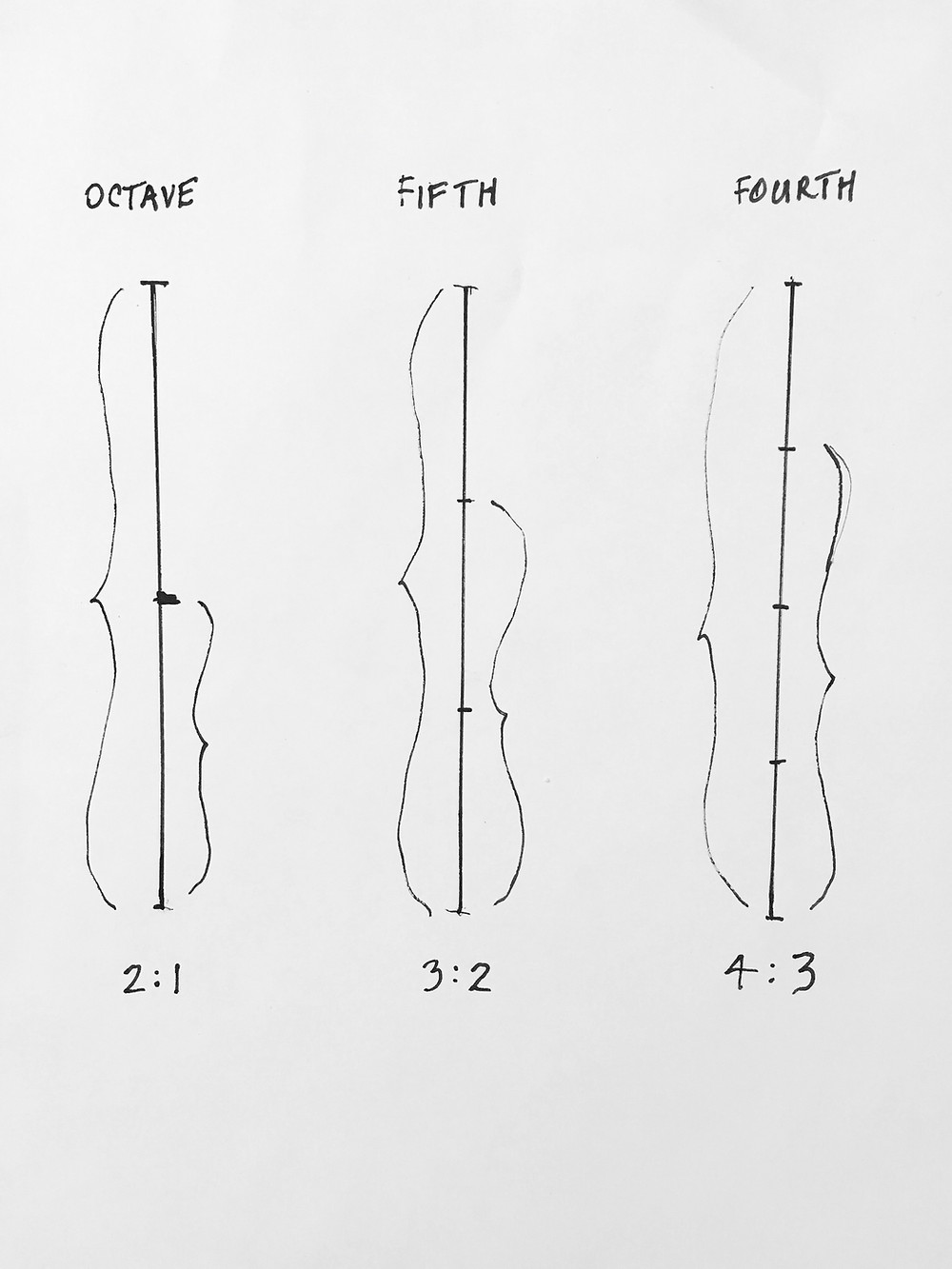Search

# Music and Mathematics

In the 6th century BC, the Greek philosopher Pythagoras discovered that the beautiful musical relationships of the Octave, the Perfect Fifth, and the Perfect Fourth were also beautiful mathematical relationships.

The Octave fraction is 2:1 - in the song "Somewhere Over the Rainbow", the first interval is a perfect octave. A Perfect Fifth fraction is 3:2 - in the song "Twinkle, Twinkle Little Star", the first interval is a perfect fifth.

A Perfect Fourth fraction is 4:3 - in the song "Here Comes the Bride", the first interval is a perfect fourth.

That simple ratios produce harmonious sounds is proof of a hidden order in the natural world, and that order is made of numbers, a profound insight that mathematicians and scientists continue to explore to this day. Every musical interval can be identified as a mathematical fraction, and mathematics is at the basis of all music. That's why a perfect interval is so pleasing to the ear, because it represents an exact mathematical ratio.Perfect Musical Intervals